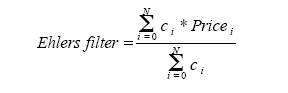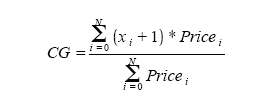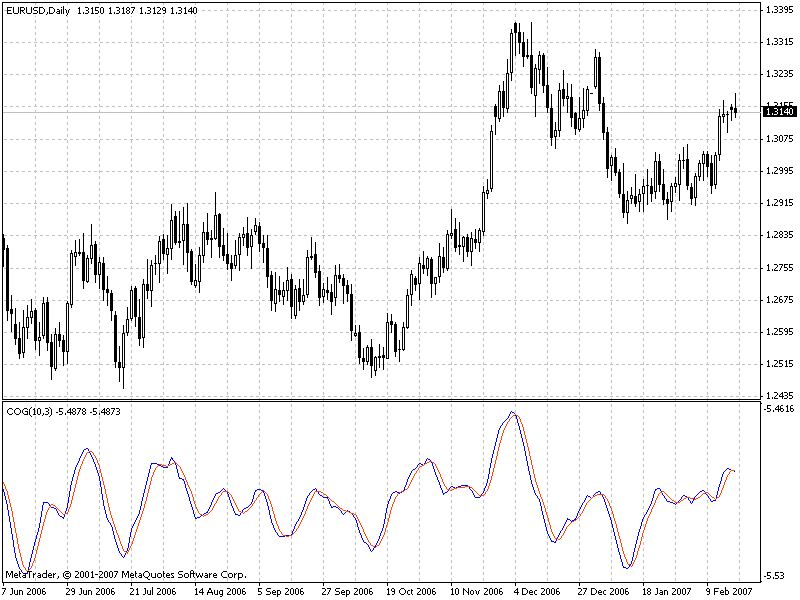Watch how to download trading robots for free
Interesting script?
So post a link to it -
let others appraise it
You liked the script? Try it in the MetaTrader 5 terminal# Center of Gravity by J. F. Ehlers - indicator for MetaTrader 4

Views:
42494
Rating:
votes: 19
Published:
2008.02.10 14:48
Updated:
2014.04.21 14:52
Center of Gravity actually has a zero lag and allows to define turning points precisely. This indicator is the result of Ehler's study of adaptive filters.

The indicator Center of Gravity allows to identify main pivot points almost without any lag. The idea of calculating a center of gravity appeared from the investigation of lags of different filters with the finite impulse response (FIR) in accordance with the relative amplitude of filter coefficients. SMA (Simple Moving Average) is a FIR-filter, in which all coefficients have one and the same value. As a result the center of gravity of SMA is an exact center of the filter. WMA (Weighted Moving Average) is a FIR-filter, in which the last price change is weighted through the filter length, and so on.

The values of weighting are coefficients of filters. Coefficients of WMA filters can be presented as contours of a triangle. The center of gravity is on the 1/3 of the triangle base length. Thus WMA gravity center is shifted to the right with respect to the center of gravitation of SMA of the same length, which gives us a smaller lag. For all examples with FIR filters the sum of productions of coefficients and the price must be divided by the sum of coefficients for preservation of original prices.

The most famous of such FIR filters is Ehlers filter that can be presented the following way:The Center of Gravity is calculated as Ehlers filter using the formula:In this indicator the parameter Per=10 sets the period for the indicator calculation, the parameter PriceType=0 sets the price type, based on which the indicator is calculated - thus we get the main line (blue color). For the signal line (red color) the parameter SmoothPer=3 sets the period of smoothing the main indicator line, the parameter SmoothType=0 denotes the type of smoothing. The interpretation of the values of parameters is given in the form of comments in the indicator code.

Translated from Russian by MetaQuotes Software Corp.
Original code: https://www.mql5.com/ru/code/7068Spearman's Rank Correlation

Spearman's Rank Correlation is a non-parametric method used in order to make statistical studies of relations between phenomena. In this case, the factual degree of parallelism between two numeric sequences will be detected.Smoothed ADX by John Ehlers

There are many algorithms of smoothing. This given indicator is for smoothing of standard indicator ADX. The code was translated from Easy Language.NRTR GATOR

It is NRTR indicator, in which candlesticks are painted in accordance with the mutual positions of Alligator lines.HVR

HVR indicator.